### Ordinary Differential Equations: Solving Systems of IVPs

### Solving Systems of IVPs

Many engineering problems contain systems of IVPs in which more than one dependent variable is a function of one independent variable, say. For example, chemical reactions of more than one component are usually described using systems of IVPs in which the initial conditions are known. Similarly, the interaction of biological growth of different species (predators and prey) is usually represented by a system of IVPs in which the initial conditions are given. Generally, these systems can be represented as: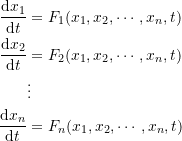The independent variable is discretized such that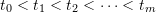with a constant step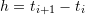. In such systems, the initial conditions, namely the values of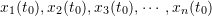, are given. The values of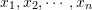when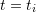are denoted: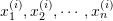. In this case, the values of the dependent variables at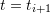can be obtained using the following system of equations: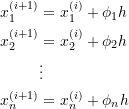where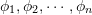depend on the method used. For example, if the explicit Euler method is used, then: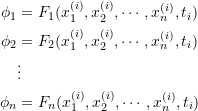Alternatively, if the classical Runge-Kutta method is used, then: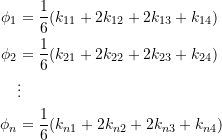where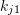,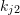,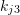, and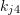are the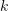values associated with the dependent variable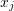and calculated according to the classical Runge-Kutta technique.

It should also be noted that higher-order IVPs can be solved by converting them into a system of first-order IVPs. For example, consider the IVP: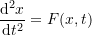with the initial conditions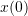and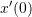given. Then, setting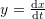, the equation can be written as a system of two IVPs: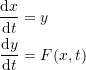with initial conditions given forand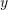.

#### Example

Consider the damped spring-mass mechanical system with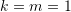,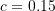with the initial conditions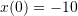and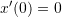. Find the numerical solution for the position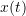and the velocity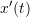for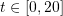using the explicit Euler method and the Runge-Kutta method. Use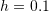.

##### Solution

The differential equation of the damped system is given by: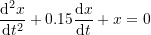The exact solution can be obtained analytically as: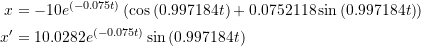Notice that this is a second order ODE. To find the numerical solution, the equation will be converted into a system of 2 IVPs. Setting, the equation can be converted into the following system:with the initial conditionsand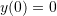. With, the interval can be split into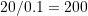time steps. The time discretization will be such that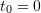,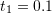,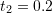, up to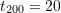. The corresponding positions are given by: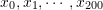while the corresponding velocities are given by: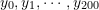.

##### Explicit Euler Method

Using the explicit Euler method, the values of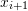and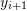can be evaluated as: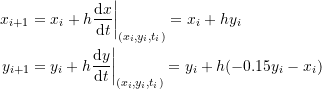With the initial conditionsand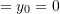, the values of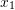and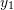atcan be calculated as: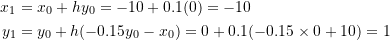Similarly, the values of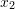and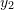can be obtained as: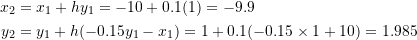Proceeding iteratively, the values ofand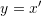up to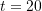can be obtained. The following figure shows the position (top) and velocity (bottom) obtained with the Explicit Euler method with(black dots) compared to the exact solution (blue line). The Microsoft Excel file EulerSystem.xlsx shows the obtained values.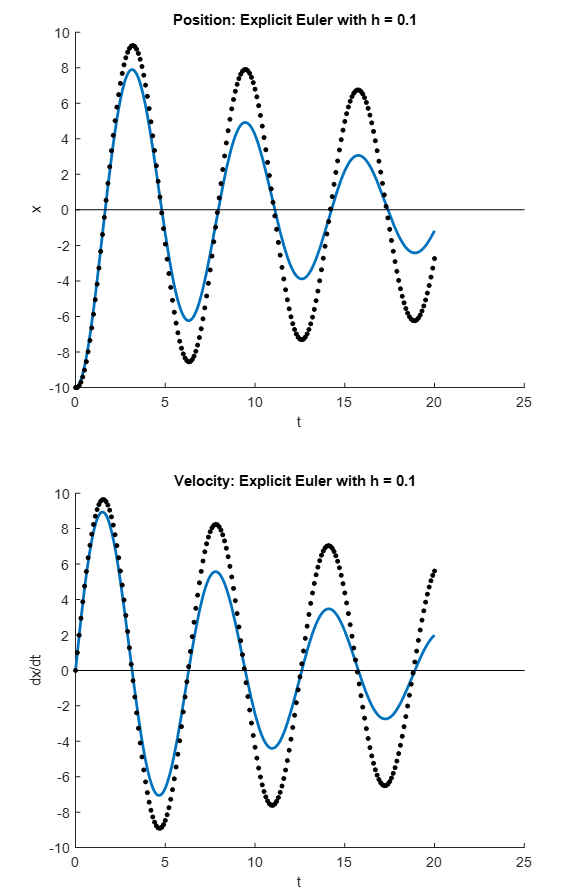##### Classical Runge-Kutta method

The two differential equations are designated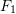and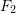as follows: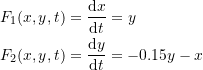Using the classical Runge-Kutta method, the values ofandcan be evaluated as: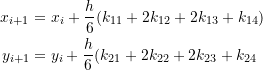where: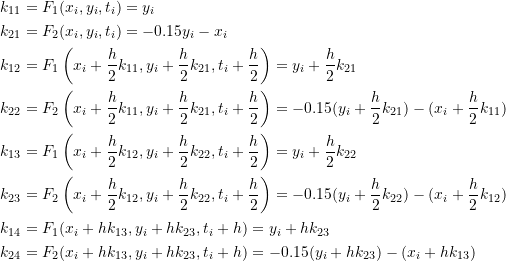With the initial conditions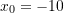and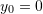, the values of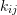can be calculated as: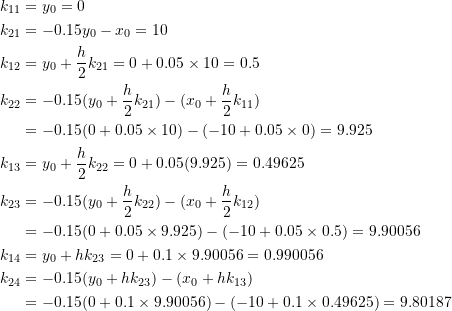Therefore: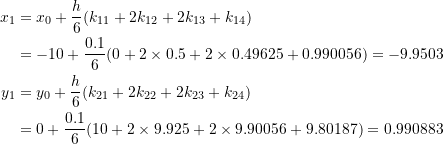Proceeding iteratively, the values ofandup tocan be obtained. The following figure shows the position (top) and velocity (bottom) obtained with the classical Runge-Kutta method with(black dots) compared to the exact solution (blue line). The Microsoft Excel file RK4System.xlsx shows the calculated up to the required time point.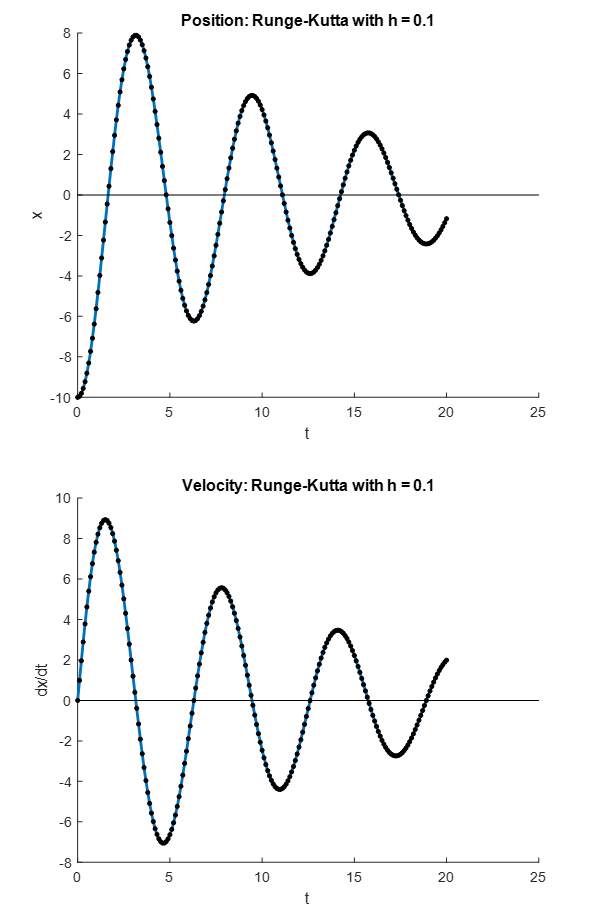##### Comparison

From the figures above, it is evident that using the explicit Euler method, the numerical solution deviates from the exact solution for higher values of. However, the classical Runge-Kutta method provides very accurate predictions.

As shown in the figure below, the Explicit Euler method can produce results with higher accuracy using smaller values of the step size (i.e.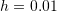). With higher values of the step size, the method can become unstable and grow without bound (i.e.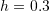).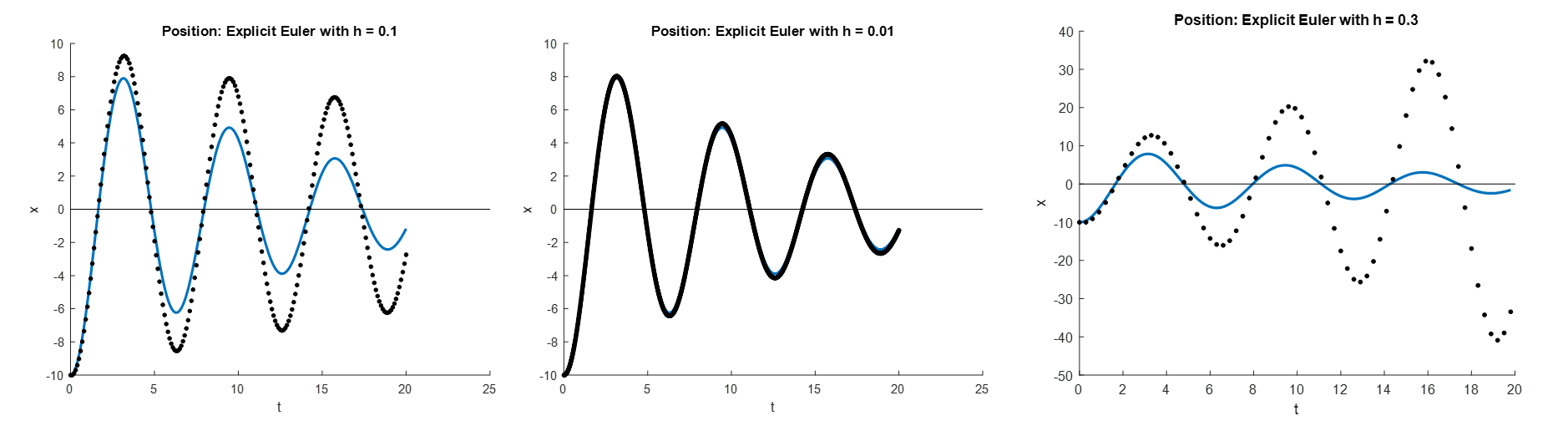In contrast, the figure below demonstrates that the classical Runge-Kutta method is able to produce accurate results and maintain numerical stability for a step size as high as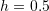.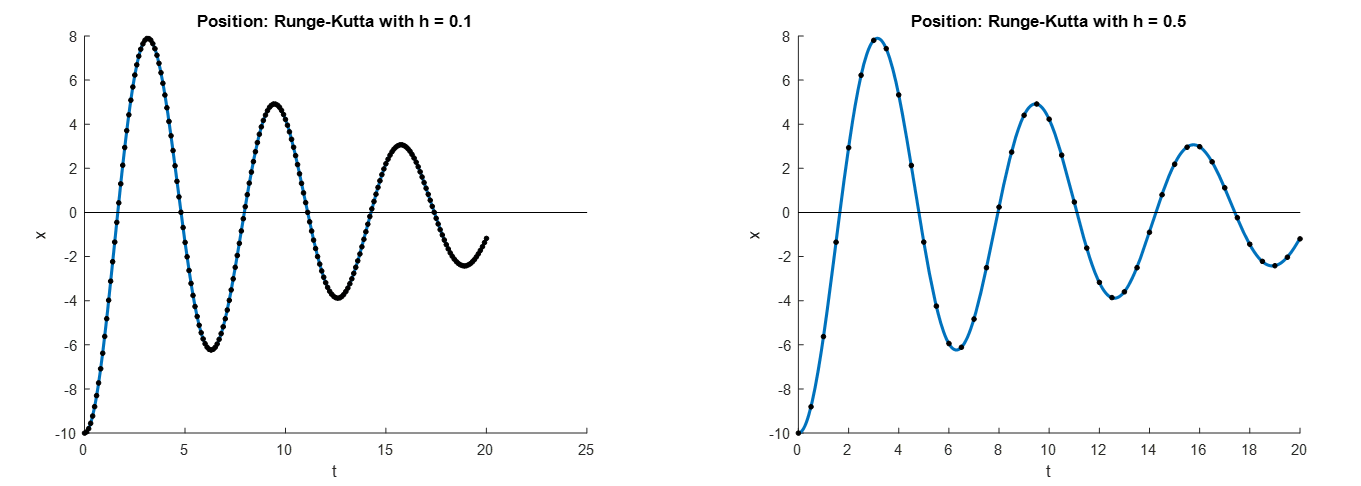The Mathematica code utilized to produce the curves is shown below.

View Mathematica Code

Clear[x]
a = DSolve[{Derivative[x][t] == -(0.15*Derivative[x][t]) - x[t], Derivative[x] == 0, x == -10}, x, t]
x = x[t] /. a[]
xp = Simplify[D[x, {t}]]

Plot[x, {t, 0, 20}, AxesOrigin -> {0, 0}, AxesOrigin -> {0, 0}, AxesLabel -> {"time", "x (position)"}]

Plot[xp, {t, 0, 20}, AxesOrigin -> {0, 0}, AxesOrigin -> {0, 0}, AxesLabel -> {"time", "x' (velocity)"}]

EulerMethod2[fp1_, fp2_, x10_, x20_, h_, t0_, tmax_] := (n = (tmax - t0)/h + 1; x1table = Table[0, {i, 1, n}]; x2table = Table[0, {i, 1, n}]; x1table[] = x10; x2table[] = x20;
Do[x1table[[i]] = h*fp1[x1table[[i - 1]], x2table[[i - 1]], h*(i - 2) + t0] + x1table[[i - 1]]; x2table[[i]] = h*fp2[x1table[[i - 1]], x2table[[i - 1]], h*(i - 2) + t0] +
x2table[[i - 1]], {i, 2, n}]; Data = Table[{h*(i - 1) + t0, x1table[[i]], x2table[[i]]}, {i, 1, n}]; Data)

RK4Method2[fp1_, fp2_, x10_, x20_, h_, t0_, tmax_] := (n = (tmax - t0)/h + 1; x1table = Table[0, {i, 1, n}]; x1table[] = x10; x2table = Table[0, {i, 1, n}]; x2table[] = x20;
Do[k11 = fp1[x1table[[i - 1]], x2table[[i - 1]], h*(i - 2) + t0]; k21 = fp2[x1table[[i - 1]], x2table[[i - 1]], h*(i - 2) + t0];
k12 = fp1[(h*k11)/2 + x1table[[i - 1]], (h*k21)/2 + x2table[[i - 1]], h*(i - 1.5) + t0]; k22 = fp2[(h*k11)/2 + x1table[[i - 1]], (h*k21)/2 + x2table[[i - 1]], h*(i - 1.5) + t0];
k13 = fp1[(h*k12)/2 + x1table[[i - 1]], (h*k22)/2 + x2table[[i - 1]], h*(i - 1.5) + t0]; k23 = fp2[(h*k12)/2 + x1table[[i - 1]], (h*k22)/2 + x2table[[i - 1]], h*(i - 1.5) + t0];
k14 = fp1[h*k13 + x1table[[i - 1]], h*k23 + x2table[[i - 1]], h*(i - 1) + t0]; k24 = fp2[h*k13 + x1table[[i - 1]], h*k23 + x2table[[i - 1]], h*(i - 1) + t0];
x1table[[i]] = (1/6)*h*(k11 + 2*k12 + 2*k13 + k14) + x1table[[i - 1]]; x2table[[i]] = (1/6)*h*(k21 + 2*k22 + 2*k23 + k24) + x2table[[i - 1]], {i, 2, n}];
Data2 = Table[{h*(i - 1) + t0, x1table[[i]], x2table[[i]]}, {i, 1, n}]; Data2)

fp1[x_, y_, t_] := y;
fp2[x_, y_, t_] := -x - 0.15*y;
Data = EulerMethod2[fp1, fp2, -10, 0, 0.1, 0, 20];

DataPosition = Drop[Data, None, {3}];
DataVelocity = Drop[Data, None, {2}];
a1 = Plot[x, {t, 0, 20}, Epilog -> {PointSize[Large], Point[DataPosition]}, AxesOrigin -> {0, 0}, ImageSize -> Medium, AxesLabel -> {"Time", "x (position)"}];
a2 = Plot[xp, {t, 0, 20}, Epilog -> {PointSize[Large], Point[DataVelocity]}, AxesOrigin -> {0, 0}, ImageSize -> Medium, AxesLabel -> {"Time", "x' (velocity)"}];
a = Grid[{{a1, a2}}];
Grid[{{"Explicit Euler"}, {a}}]
Data = RK4Method2[fp1, fp2, -10, 0, 0.1, 0, 20];
DataPosition = Drop[Data, None, {3}];
DataVelocity = Drop[Data, None, {2}];
a1 = Plot[x, {t, 0, 20}, Epilog -> {PointSize[Large], Point[DataPosition]}, ImageSize -> Medium, AxesOrigin -> {0, 0}, AxesLabel -> {"Time", "x (position)"}];
a2 = Plot[xp, {t, 0, 20}, Epilog -> {PointSize[Large], Point[DataVelocity]}, ImageSize -> Medium, AxesOrigin -> {0, 0}, AxesLabel -> {"Time", "x' (velocity)"}];
a = Grid[{{a1, a2}}];
Grid[{{"Classical Runge-Kutta"}, {a}}]

View Python Code
# UPGRADE: need Sympy 1.2 or later, upgrade by running: "!pip install sympy --upgrade" in a code cell
import numpy as np
import sympy as sp
import matplotlib.pyplot as plt
sp.init_printing(use_latex=True)

x = sp.Function('x')
t = sp.symbols('t')
sol = sp.dsolve(x(t).diff(t,2) + x(t) + 0.15*x(t).diff(t), ics={x(0): -10, x(t).diff(t).subs(t,0): 0})
display(sol)
xp = sp.simplify(sol.rhs.diff(t))
display(xp)

x_val = np.arange(0,20,0.01)
plt.plot(x_val, [sol.subs(t, i).rhs for i in x_val])
plt.xlabel("time"); plt.ylabel("x (position)")
plt.grid(); plt.show()
plt.plot(x_val, [xp.subs(t, i) for i in x_val])
plt.xlabel("time"); plt.ylabel("x' (velocity)")
plt.grid(); plt.show()

def EulerMethod2(fp1, fp2, x10, x20, h, t0, tmax):
n = int((tmax - t0)/h + 1)
x1table = [0 for i in range(n)]
x2table = [0 for i in range(n)]
x1table = x10
x2table = x20
for i in range(1,n):
x1table[i] = x1table[i - 1] +  h*fp1(x1table[i - 1], x2table[i - 1], t0 + (i - 1)*h)
x2table[i] = x2table[i - 1] +  h*fp2(x1table[i - 1], x2table[i - 1], t0 + (i - 1)*h)
Data = [[t0 + i*h, x1table[i], x2table[i]] for i in range(n)]
return Data

def RK4Method2(fp1, fp2, x10, x20, h, t0, tmax):
n = int((tmax - t0)/h + 1)
x1table = [0 for i in range(n)]
x1table = x10
x2table = [0 for i in range(n)]
x2table = x20
for i in range(1,n):
k11 = fp1(x1table[i - 1], x2table[i - 1], t0 + (i - 1)*h)
k21 = fp2(x1table[i - 1], x2table[i - 1], t0 + (i - 1)*h)
k12 = fp1(x1table[i - 1] + h/2*k11, x2table[i - 1] + h/2*k21, t0 + (i - 0.5)*h)
k22 = fp2(x1table[i - 1] + h/2*k11, x2table[i - 1] + h/2*k21, t0 + (i - 0.5)*h)
k13 = fp1(x1table[i - 1] + h/2*k12, x2table[i - 1] + h/2*k22, t0 + (i - 0.5)*h)
k23 = fp2(x1table[i - 1] + h/2*k12, x2table[i - 1] + h/2*k22, t0 + (i - 0.5)*h)
k14 = fp1(x1table[i - 1] + h*k13, x2table[i - 1] + h*k23, t0 + i*h)
k24 = fp2(x1table[i - 1] + h*k13, x2table[i - 1] + h*k23, t0 + i*h)
x1table[i] = x1table[i - 1] + h*(k11 + 2*k12 + 2*k13 + k14)/6
x2table[i] = x2table[i - 1] + h*(k21 + 2*k22 + 2*k23 + k24)/6
Data = [[t0 + i*h, x1table[i], x2table[i]] for i in range(n)]
return Data

def fp1(x, y, t): return y
def fp2(x, y, t): return -x - 0.15*y
x_val = np.arange(0,20,0.01)

Data = EulerMethod2(fp1, fp2, -10, 0, 0.1, 0, 20)
plt.title("Explicit Euler")
plt.plot(x_val, [sol.subs(t, i).rhs for i in x_val])
plt.scatter([DataPosition for DataPosition in Data],
[DataPosition for DataPosition in Data],c='k')
plt.xlabel("Time"); plt.ylabel("x (position)")
plt.grid(); plt.show()
plt.title("Explicit Euler")
plt.plot(x_val, [xp.subs(t, i) for i in x_val])
plt.scatter([DataVelocity for DataVelocity in Data],
[DataVelocity for DataVelocity in Data],c='k')
plt.xlabel("Time"); plt.ylabel("x' (velocity)")
plt.grid(); plt.show()

Data = RK4Method2(fp1, fp2, -10, 0, 0.1, 0, 20)
plt.title("Classical Runge-Kutta")
plt.plot(x_val, [sol.subs(t, i).rhs for i in x_val])
plt.scatter([DataPosition for DataPosition in Data],
[DataPosition for DataPosition in Data],c='k')
plt.xlabel("Time"); plt.ylabel("x (position)")
plt.grid(); plt.show()
plt.title("Classical Runge-Kutta")
plt.plot(x_val, [xp.subs(t, i) for i in x_val])
plt.scatter([DataVelocity for DataVelocity in Data],
[DataVelocity for DataVelocity in Data],c='k')
plt.xlabel("Time"); plt.ylabel("x' (velocity)")
plt.grid(); plt.show()


The effect of changing the value of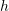on the resulting numerical solution for the system of IVPs given here can be illustrated using the following tool. Even forthe classical Runge-Kutta produces very accurate predictions at which point the explicit Euler method produces very erroneous results.

#### Using Mathematica

The built-in NDSolve in Mathematica uses the Runge-Kutta methods to solve a system of IVPs and produces piecewise interpolating polynomials for the dependent variables. The following code solves some of the preceding examples and compares with the exact solution. Note that in those examples when the exact and numerical solutions are plotted together, they overlap!

View Mathematica Code
Clear[x, xn, xtable]
(*Example 1*)
k = 0.015;
a = DSolve[{x'[t] == k*x[t], x == 35}, x, t];
x = x[t] /. a[]

b = NDSolve[{xn'[t] == k*xn[t], xn == 35}, xn, {t, 0, 50}]
xn = xn /. b[]
Plot[{x, xn[t]}, {t, 0, 50}, PlotLegends -> {"Exact", "Numerical using Mathematica"}, AxesLabel -> {"t", "Population"}]

(*Example 2*)
Clear[cn]
b = NDSolve[{cn'[t] == (100 + 50*Cos[2 Pi*t/365])/10000*(5 Exp[-2 t/1000] - cn[t]), cn == 5}, cn, {t, 0, 700}]
cn = cn /. b[]
Plot[cn[t], {t, 0, 700}, AxesLabel -> {"t", "Concentration"}]

(*Example 4*)
Clear[xn, x]
a = DSolve[{x'[t] == t*x[t]^2 + 2*x[t], x == -5}, x, t];
x = x[t] /. a[]
b = NDSolve[{xn'[t] == t*xn[t]^2 + 2 xn[t], xn == -5},  xn, {t, 0, 5}]
xn = xn /. b[]
Plot[{x, xn[t]}, {t, 0, 5}, PlotRange -> All, PlotLegends -> {"Exact", "Numerical using Mathematica"}, AxesLabel -> {"t", "x"}]

(*Example of a system*)
Clear[xn, yn, x]
a = DSolve[{x''[t] == 10 - x[t] - 0.15 x'[t], x' == 0, x == 0},   x, t]
x = x[t] /. a[]
xp = Simplify[D[x, t]]
b = NDSolve[{xn'[t] == yn[t], yn'[t] == 10 - xn[t] - 0.15 yn[t],    xn == 0, yn == 0}, {xn, yn}, {t, 0, 2 Pi}]
xn = xn /. b[]
yn = yn /. b[]

Plot[{x, xn[t]}, {t, 0, 2 Pi}, AxesOrigin -> {0, 0},  AxesOrigin -> {0, 0},  PlotLegends -> {"Exact", "Numerical using Mathematica"},  AxesLabel -> {"time", "x (position)"}]
Plot[{xp, yn[t]}, {t, 0, 2 Pi}, AxesOrigin -> {0, 0},  AxesOrigin -> {0, 0},  PlotLegends -> {"Exact", "Numerical using Mathematica"},  AxesLabel -> {"time", "x' (velocity)"}]


View Python Code
# UPGRADE: need Sympy 1.2 or later, upgrade by running: "!pip install sympy --upgrade" in a code cell
import numpy as np
import sympy as sp
import matplotlib.pyplot as plt
from scipy.integrate import odeint
sp.init_printing(use_latex=True)

x = sp.Function('x')
t, k = sp.symbols('t k')

# Example 1
k = 0.015
a = sp.dsolve(x(t).diff(t) - k*x(t), ics={x(0): 35})
display(a)
def f(x, t, params):
k = params
dxdt = k*x
return dxdt
x_val = np.arange(0,50,0.1)
b = odeint(f, , x_val, args=(k,))
plt.plot(x_val, [a.subs(t, i).rhs for i in x_val], label="Exact")
plt.plot(x_val, b, label="Numerical")
plt.xlabel("t"); plt.ylabel("Population")
plt.legend(); plt.grid(); plt.show()

# Example 2
def f(c, t):
dcdt = (100 + 50*np.cos(2*np.pi*t/365))/10000*(5*np.exp(-2*t/1000) - c)
return dcdt
x_val = np.arange(0,700,0.1)
b = odeint(f, , x_val)
plt.plot(x_val, b)
plt.xlabel("t"); plt.ylabel("Concentration")
plt.grid(); plt.show()

# Example 4
a = sp.dsolve(x(t).diff(t) - t*x(t)**2 - 2*x(t), ics={x(0): -5})
display(a)
def f(x, t):
dxdt = t*x**2 + 2*x
return dxdt
x_val = np.arange(0,5,0.1)
b = odeint(f, [-5], x_val)
plt.plot(x_val, [a.subs(t, i).rhs for i in x_val], label="Exact")
plt.plot(x_val, b, label="Numerical")
plt.xlabel("t"); plt.ylabel("x")
plt.legend(); plt.grid(); plt.show()

# Example of a system
a = sp.dsolve(x(t).diff(t,2) - 10 + x(t) + 0.15*x(t).diff(t), ics={x(t).diff(t).subs(t,0): 0, x(0): 0})
ap = sp.diff(a.rhs,t)
display(a)
display(ap)
def f(vars, t):
x, y = vars
dxdt = y
dydt = 10 - x - 0.15*y
return [dxdt, dydt]
x_val = np.arange(0,2*np.pi,0.1)
b = odeint(f, [0,0], x_val)
xn = b[:, 0]
yn = b[:, 1]

plt.plot(x_val, [a.subs(t, i).rhs for i in x_val], label="Exact")
plt.plot(x_val, xn, label="Numerical")
plt.xlabel("Time"); plt.ylabel("x (position)")
plt.legend(); plt.grid(); plt.show()

plt.plot(x_val, [ap.subs(t, i) for i in x_val], label="Exact")
plt.plot(x_val, yn, label="Numerical")
plt.xlabel("Time"); plt.ylabel("x' (velocity)")
plt.legend(); plt.grid(); plt.show()